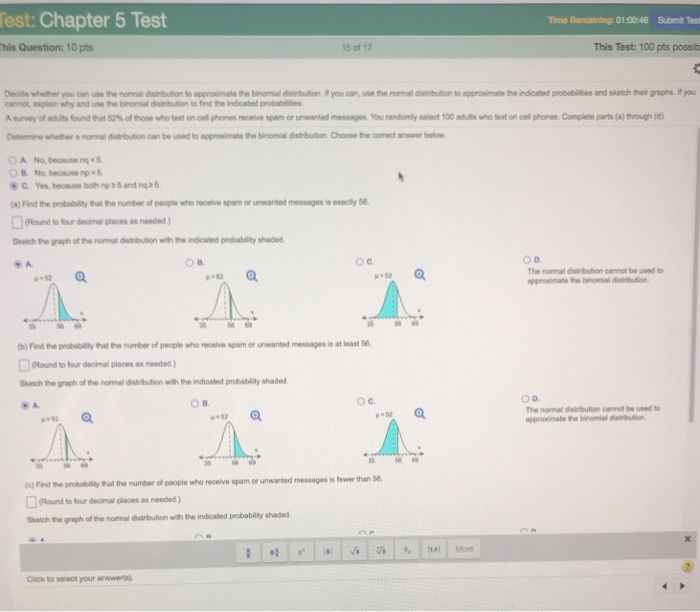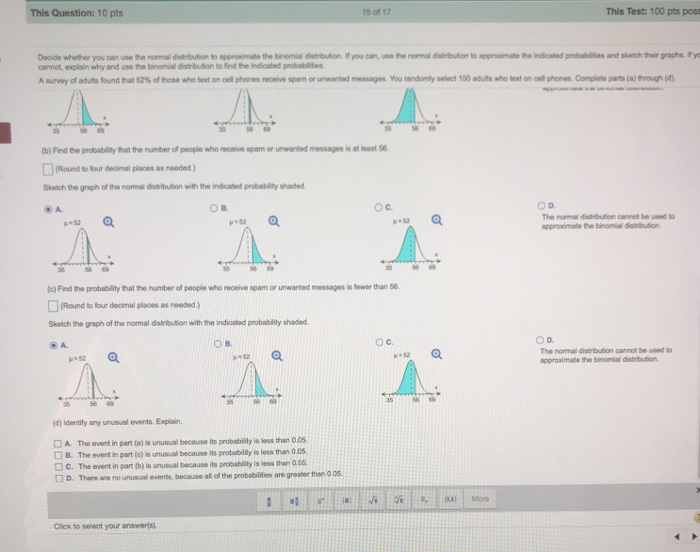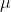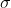# est: Chapter 5 Test Time Remaining: 01:00:46 Submilt Test his Question: 10 pts 15 of 17...est: Chapter 5 Test Time Remaining: 01:00:46 Submilt Test his Question: 10 pts 15 of 17 This Test: 100 pts possib Decide whether you can use the normal distthion to approinate the bironnaldstrbnon 냈you can use the normal distritton to apprainate te ideated pobabites ausetter gats if you cannot, explain why and use the binomial distribution to find the indicated probabilities Asurvey of Butsfound that 52% of tose who text on cel phones receive spam or unanted mete·You rantmiysted 00 ad Stohtonce. phone. Co peessa) men is. Determine whether a nomal distribution can be used to approximale the binomial distribution Choose the correct answer below A. No, because ng «s C.Yesbecause both np5 and no a6 (a)Find the probability that the number of people who receive spam or unwanted messages is exacy 56 □(Round toRx, decirw places as needed) Sketch th ghofthe norrmal dstrit don we'the indicated probability shaded c. The normal distribution cannot be used to approximale the binomial disirbution 52 35 4 3 tb) Find the probability that the number of people who receive spam or unwanted messages is at least 56 □(Round to for decrnal places as needed) kelch the graph of the normal distribution with the indioaled probability shaded O B. OC. The normal bution cainot be used to ,.52 Ps52 μ-52 e) Find the probabilly hat the number of people who receive spam or unwarted messages i tewer than 5 □(Round to tur deornal places as needed ) Skatch the graph of the normal distribution with the indicated probability shaded Click to select your anawerls).= n * p = 100 * 0.52 = 52= sqrt(n * p * (1 - p)) = sqrt(100 * 0.52 * 0.48) = 5

a) P(X = 56) = P(56 < X < 56)

= P(55.5 < X < 56.5)

= P((55.5 -)/< ( X -)/< (56.5 -)/)

= P((55.5 - 52)/5 < Z < (56.5 - 52)/5)

= P(0.7 < Z < 0.9)

= P(Z < 0.9) - P(Z < 0.7)

= 0.8159 - 0.7580 = 0.0579

Option-B is correct graph.

b) P(X > 56) = P(56 < X)

= P(55.5 < X)

= P((55.5 -)/< ( X -)/)

= P((55.5 - 52)/5 < Z)

= P(0.7 < Z)

= 1 - P(Z < 0.7)

= 1 - 0.7580 = 0.242

Option - B is correct graph.

P(X < 56) = P(( X -)/< (56.5 -)/)

= P(Z < (56.5 - 52)/5)

= P(Z < 0.9)

= 0.8159

Option-c is the correct graph.

D) Option- D is the correct answer

#### Earn Coin

Coins can be redeemed for fabulous gifts.